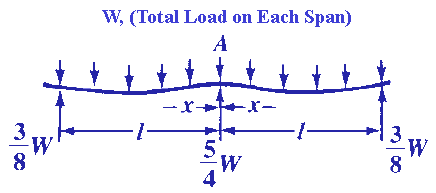Bending, Deflection and Stress Equations Calculator for
Continuous Beam, with Two Equal Spans, Uniform Load

Beam Deflection and Stress Formula and Calculators

Structural Beam Deflection, Stress, Bending Equations and calculator for a ontinuous Beam, with Two Equal Spans, Uniform Load.Section Modulus Z

Z = I/c

I = Moment of inertia Cross Section : Units in4 (m4)
z = Distance neutral axis to extreme fiber: Units in (m)

General Stress at any Given PointDeflection at any PointCritical DeflectionsWhere:

 E = Modulus of Elasticity psi (N/mm2) I = Moment of Inertia in4 (mm4) W = Load lbs (N) s = Stress at the cross-section being evaluated Lbs/in2 (N/mm2) y = Deflection inches (mm) x = Some distance as indicated inches (mm) u = Some distance as indicated inches (mm) a, b = Some distance as indicated inches (mm) L = Some distance as indicated inches (mm)= Some distance as indicated inches (mm) Z = section modulus of the cross-section of the beam = I/z in3 (mm3) z = distance from neutral axis to extreme fiber (edge) inches (mm)
• Please note letter "" (lower case "L") is different than "I" (Moment of Inertia).
• Deflections apply only to constant cross sections along entire length.

References:

• Any Machinery's Handbook published since 1931 or,
• Machinery's Handbook, 21st Edition
• Machinery's Handbook, 23st Edition
• Machinery's Handbook, 27st Edition© Copyright 2000 - 2019, by Engineers Edge, LLC www.engineersedge.comMembership Register | LoginHomeEngineering Book StoreEngineering ForumExcel App. DownloadsOnline Books & ManualsEngineering NewsEngineering VideosEngineering CalculatorsEngineering ToolboxGD&T Training Geometric Dimensioning TolerancingDFM DFA TrainingTraining Online EngineeringAdvertising CenterCopyright Notice